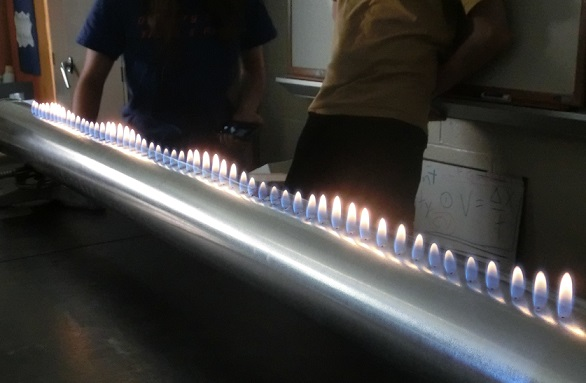# Standing Waves Demonstrations

2 teachers like this lesson
Print Lesson

## Objective

Students observe the application of standing waves as they form in a Rubens' tube.

#### Big Idea

Standing waves result from wave interference and wave behavior and apply to all types of waves.

## Context and Supplies

Today, students review the homework questions assigned during the previous class, Standing Waves. Then they witness the application of standing waves that form on a Reubens' tube when the right frequency is played. Due to standardized state testing, we have a half-day today so the class period is only 25 minutes long.

Students apply CCSS Math Practice 8: Look for and express regularity in repeated reasoning as they view a standing wave form with air and sound waves instead of a string. Also applied is NGSS Science Practice 2: Developing and using models and Science Practice 6: Constructing explanations. Students calculate the speed of sound in propane at the end of the lesson which applies Science Practice 5: Using mathematics and computational thinking.

The NGSS Performance standard which this lesson uses is HS-PS4-5: Communicate technical information about how some technological devices use the principles of wave behavior and wave interactions with matter to transmit and capture information and energy as the focus of the lesson is on the creation of standing waves which is a direct result of wave behavior, specifically wave interference and reflection off a barrier.

## Homework Review of Wave Big Ideas

10 minutes

Students did a homework assignment which is a Socrative quiz titled Intro to Waves - Big Ideas.  Socrative is a great tool that allows students to answer questions with their smart phones or computers and the program grades the results for the teacher. In this case, I enter the assignment as a homework grade even through Socrative refers to the assignments as a quiz. I set the quiz to scramble the questions and answers and to not reveal the answers as the students take the quiz; this is done to reduce the incidence of students supplying solutions to one another.

We start the class with a review of the homework. I use my document camera to project the Intro to Waves - Big Ideas Solutions solutions and call on random students to read each of the problems (preview mode only shows the first page, download the PDF to see the complete document).   The goal of this homework is to reinforce the big ideas of the unit that have been explored so far. Those are:

1. The velocity of a wave is determined by the medium.

2. The frequency of a wave is determined by the wave source.

3. The wavelength is a result of the frequency and velocity according to the wave equation.

4. The amplitude of a wave is determined by the energy of a wave.

5. The amplitudes of separate waves add together when waves interfere.

## Standing Wave Demonstration

15 minutes

After we review the big ideas of the wave unit, it is time for a demonstration. I fire up the Rubens' tube, which is a hollow metal tube closed at both ends and has a gas supply line attached to it. A series of 1 mm holes are along the length of the tube about 2 cm apart which allows the gas to escape. This gas is set on fire.One of the closed ends is a rubber membrane (a stretched balloon). With a speaker pointed at the end with the membrane, we can make the flames along the top do a variety of interesting things based on the sound put through the tube. I start off with the Gangnam Style parody, NASA Johnson Style. It is an appropriate song for physics class and is a good song to get the flames to dance. I show students that there is a speaker at one end I ask questions of the class such as "Why do the flames move?", "What happens when there is a pause in the music?", "What types of sounds make the flames move the most?" These questions are designed to help the students make the connection between sound waves and air pressure and how it is the increased pressure that causes the flames to jump.

Then I turn off the music and play a tone using a website called Online Tone Generator. This allows me to start at a low frequency, such as 50 Hz, and then slowly increase the frequency. There is a mild effect on the flames for the frequencies from 50-400 Hz, but then right around 450 Hz standing waves begin to form on the tube.As I continue through the frequencies, you can see different wavelength standing waves form, the wavelength gets smaller with increasing frequencies. I then display a DIY web page called Instructables - The Rubens' Tube. This website has a great overview a Rubens' Tube and shows how the standing wave that form in the tube creates the flame pattern that we see.

In the final few minutes, I have students apply mathematical thinking to the demonstration. I ask students to get out a piece of paper and to calculate the speed of sound in propane (the gas that is in the tube). I read the value on the tone generator and tell the students that the frequency is 450 Hz. Then I use a meter stick to carefully measure the distance from one flame peak to the next and report that as 0.54 meters. I don't tell the students this, but I expect them to know they should use the wave equation (velocity = frequency * wavelength) to calculate a velocity of 245 m/s.  As students leave, I collect their calculations to assess them for a grade.